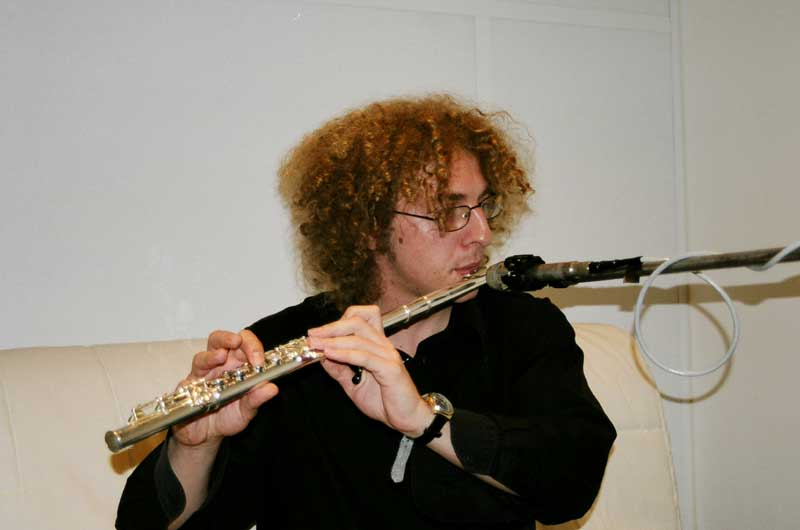Libor BartoDOMU VYZKUM PRO STUDENTY

ARCHIV 13/14 letni semestr

[Zpet]

LINEARNI ALGEBRA A GEOMETRIE 2 (NMAG102)

UNIVERSAL ALGEBRA 2 (NMAG450)

Lecture: Thursday 15:40 - 17:10 KA
Tutorial: Thursday 17:20 - 18:05 KA (instructor: Jakub Oprsal)

LECTURES

• 27.2. Malt'sev conditions for congruence permutability and congruence distributivity.
• 6.3. Interpretation, interpretability lattice. Thm: Idempotent variety V is not interpretable into sets iff V has a Taylor term.
• 13.3. Equational theory. Thm: Id(Mod(E)) = eq.theory generated by E. Equational completeness theorem (semantic consequence=syntactic consequence).
• 20.3. Rewriting systems. Converegent digraphs. Thm: Convergent = locally confluent + finitely terminating. Reduction order. The most general unifier, critical pair. Knuth-Bendix algorithm.
• 27.3. Knuth-Bendix ordering.
• 3.4. Finile based variety/algebra. Thm: finitely based = finitely axiomatizable. Thm: depends only on the clone of the algebra. Thm: F.B. <=> basis of n-variable identites for some n. Example of a finite algebra which is not finitely based.
• 10.4. Definable principal congruences (DPC). Thm: DPC => principal congruence can be defined by "conservative" formula. Thm: V has DPC + finitely many SIs, all finite => V is finitely based.
• 17.4. Affine algebra (=polynomially equivalent to a module). Abelian algebra. Thm: affine <=> Maltsev and abelian. Thm: If Maltsev then abelian <=> affine <=> the Maltsev is central.
• 24.4. C(alpha, beta| delta): alpha centralizes beta modulo delta. Thm: It has the expected meaning in groups. Commutator.
• 1.5. ---
• 8.5. ---
• 15.5. Tame congruence theory: Neighborhood, miminal set, uniformity and density. Classification of minimal algebras.
• 22.5. ---

LITERATURE

SEMINAR K PROBLEMU CSP (NALG118)

ARCHIV